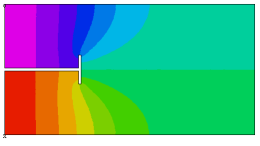﻿ Sample Problems > Usage > Eigenvalues > waveguide

# waveguide

Navigation:  Sample Problems > Usage > Eigenvalues >

# waveguide{ WAVEGUIDE.PDE

This problem solves for the Transverse-Electric modes of a T-septate

rectangular waveguide.

Assuming that Z is the propagation direction, we can write

E(x,y,z) = E(x,y)*exp(i*(omega*t-Kz*z))

H(x,y,z) = H(x,y)*exp(i*(omega*t-Kz*z))

where omega is the angular frequency and kz denotes the propagation constant.

In a Transverse-Electric waveguide, the electric field component in the propagation

direction is zero, or Ez = 0.

Substituting these equations into the source-free Maxwell's equations and rearranging,

we can write

Ey =  -(omega*mu/kz)*Hx

Ex = (omega*mu/kz)*Hy

Hx = -i*dx(Hz)*kz/kt

Hy = i*dy(Hz)*kz/kt

with kt = [omega^2*eps*mu - kz^2]

It can also be shown that in this case Hz satisfies the homogeneous Helmholtz equation

dxx(Hz) + dyy(Hz) + Kt^2*Hz = 0

together with the homogeneous Neumann boundary condition on the conducting wall

dn(Hz) = 0

In order to avoid clutter in this example script, we will supress the proportionality factors.

(The leading "i" in the definition of Hx and Hy is merely a phase shift.)

-----  From J. Jin,  "The Finite Element Method in Electromagnetics", p. 197

}

title "TE Waveguide"

select

modes = 4     { This is the number of Eigenvalues desired. }

 variables  hz   definitions  L = 2  h = 0.5       ! half box height  g = 0.01     ! half-guage of wall  s = 0.3*L     ! septum depth  tang = 0.1   ! half-width of tang  Hx = -dx(Hz)  Hy = dy(Hz)  Ex = Hy  Ey = -Hx   equationsHz:  del2(Hz) + lambda*Hz = 0       { lambda = Kt^2 }

constraints

integral(Hz) = 0 { since Hz has only natural boundary conditions,

we need an additional constraint to make

the solution unique }

boundaries

region 1

start(0,0)

natural(Hz) = 0     ! this condition applies to all subsequent segments

! walk the box body

line to (L,0) to (L,1) to (0,1) to (0,h+g)

! walk the T-septum

to (s-g,h+g) to (s-g,h+g+tang) fillet(g/2) to (s+g,h+g+tang) fillet(g/2)

to (s+g,h-g-tang) fillet(g/2) to (s-g,h-g-tang) fillet(g/2) to (s-g,h-g) to (0,h-g)

line to close

monitors

contour(Hz)

plots

contour(Hz) painted

vector(Hx,Hy) as "Transverse H" norm

contour(magnitude(Hx,Hy))

contour(magnitude(Hx,Hy)) zoom(0.5,0.5,0.2,0.2)

vector(Ex,Ey) as "Transverse E" norm

contour(magnitude(Ex,Ey))

contour(magnitude(Ex,Ey)) zoom(0.5,0.5,0.2,0.2)

end